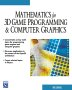# Maths - Morphism

Sometimes we can find an equivalence between operations, for instance: log(a*b) = log a + log b.## Isomorphism

If we can reverse the function in a morphism, that is if it is one to one, then it is called an isomorphism. If the function is many to one then it is a homomorphism.In the days before calculators it was quite difficult to do multiplications, so it could be easier to take the logarithm of each number, then add them together, then take the antilogarithm to get the product of the two numbers.

## Mathematical Modelling

Any application of mathematics can be though of as an isomorphism. If we cannot predict the result of a physical operation then we try to find an equivalent mathematical operation.## Morphisms within mathematics

Morphisms can be applied in:

• sets.
• groups
• vector spaces and linear transformations
• topology

## Automorphism

A morphism which preserves the symmetry. The set of automorphisms of an object together with the operation of function composition forms a group, so it has the following properties:

• Closure
• Identity
• Inverse
• Associativity## Inner and Outer automorphisms

The operation pout = m * pin *m-1 is called conjugation by m.

If m is a member of the same group as p then this is called an inner morphism, otherwise if there is no correspondence with an element of the object, it is an outer morphism.

 metadata block see also: Correspondence about this page Book Shop - Further reading. Where I can, I have put links to Amazon for books that are relevant to the subject, click on the appropriate country flag to get more details of the book or to buy it from them.Mathematics for 3D game Programming - Includes introduction to Vectors, Matrices, Transforms and Trigonometry. (But no euler angles or quaternions). Also includes ray tracing and some linear & rotational physics also collision detection (but not collision response). Other Math Books Specific to this page here:

This site may have errors. Don't use for critical systems.